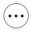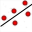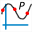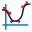Open source cross-platform software of geometry, analysis and simulation - Yves Biton

HomeIntroduction

# Version 7.0 of MathGraph32 : More adapted to statistics calculations and matricial calculations still more powerful

publication Saturday 8 January 2022.

Version 7.0 of MathGraph32 brings up these important new features :

• You can now create a matrix by import of data copied from a cellsheet.
• For this use iconat the right side of the calculations tollbar and choose item Matrix by worksheet data.
• You can now create points coordinates of which are contained in a two columns matrix, the firts coumn containing the x-coordinates and the second one containing the y-coordinates.
• For this use iconon the bottom left side of the left toolbar and choose item Set of points by 2 column atrix.
• You can now create a matrix coordinates of which are the coordinates of some points of the figure. For this use iconat the right side of the calculations tollbar and choose item Matrix of coordinates.
• First choose a name for the matrix, then click on the desired points . Click on the red button STOP to indicate you have finished.
• The created matrc is dynamic : if the points move the matrix changes with them.
• A new tool is available to create a regression line by the least squares method.
• In the toolbar of line creations, click on icon• Then click on the points used for the regression ans click on the red button STOP when you have clicked on the last point.
• To be noted : The line is created but also 2 real calculations named areg and breg giving the coefficients of the regression line.
• Matrices defined by a formula of type formula(i, j) (where i is the column index and j the row index) have now a number of rows and columns which are dynamic. This allows to create very powerful macro constructions with matrices.
• In the locuses creation toolbar, iconallows the creation of a polynomial function curve going through up to 20 points (7 in the former versions), and even with 20 points the figure remains perfectly fluid.
• The toolworks now in two modes.
• If you don’t check the checkbox Use only one polynomial function, the curve is created on each segment joining two consecutive points using a polynomial function of degree 3. It was the only mode in the former versions.
• If you check the checkbox Use only one polynomial function, the curve will be the curve of an unique polynomial function of degree 2n - 1 (or less) if you chose n points and n slopes.
• New functions are available :
• nbrow(A) where A is a matrix : returns the number of rowws of matrix A.
• May be used in a real or matricial calculation but if used in a real calculation, must be the only formula (for instance no 2*nbrow(A) in a real calculation).
• nbcol(A)where A is a matrix : returns the number of columns of matrix A.
• May be used in a real or matricial calculation but if used in a real calculation, mmust be the only formula (for instance no 2*nbcol(A) in a real calculation).
• The calculation of a matrix determinant may now be done directly in a real calculation but it must be the only formula : deter(A) will be accepted as a real calculation (where A is a matrix) but 2*deter(A) will be refused in a real calculation.
• A new real function is available :
• divmaxp(n, k) : returns the highest positive integer k
such as n is divisible by k^p with 1 < p < 256 et 1 < |n| < 1000000.
• This fonction is useful to extract a square integer from a square root.